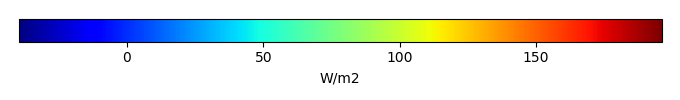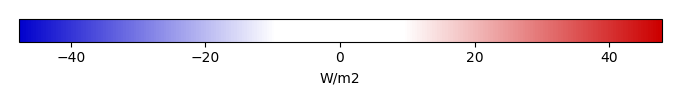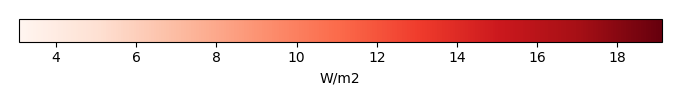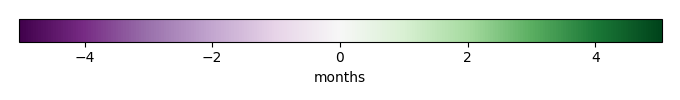# Mean State

Period Mean (original grids) [W/m2]
Model Period Mean (intersection) [W/m2]
Model Period Mean (complement) [W/m2]
Benchmark Period Mean (intersection) [W/m2]
Benchmark Period Mean (complement) [W/m2]
Bias [W/m2]
RMSE [W/m2]
Phase Shift [months]
Bias Score 
RMSE Score 
Seasonal Cycle Score 
Spatial Distribution Score 
Interannual Variability Score 
Overall Score 
Benchmark [-] 131.
CLM4 [-] 120. 121. 0.00 130. 157. -8.85 20.7 1.31 0.60 0.42 0.83 0.98 0.72 0.66
CLM4.5 [-] 117. 117. 0.00 130. 157. -12.6 22.4 1.29 0.54 0.42 0.83 1.0 0.72 0.65
CLM5 [-] 116. 116. 0.00 130. 157. -13.7 22.6 1.40 0.51 0.42 0.81 0.99 0.69 0.64
Period Mean (original grids) [W/m2]
Model Period Mean (intersection) [W/m2]
Model Period Mean (complement) [W/m2]
Benchmark Period Mean (intersection) [W/m2]
Benchmark Period Mean (complement) [W/m2]
Bias [W/m2]
RMSE [W/m2]
Phase Shift [months]
Bias Score 
RMSE Score 
Seasonal Cycle Score 
Spatial Distribution Score 
Interannual Variability Score 
Overall Score 
Benchmark [-] 123.
CLM4 [-] 103. 102. 0.00 122. 160. -19.6 26.7 1.30 0.47 0.49 0.82 1.0 0.71 0.66
CLM4.5 [-] 98.3 97.8 0.00 122. 160. -23.9 29.8 1.38 0.39 0.50 0.81 0.92 0.71 0.64
CLM5 [-] 97.4 96.9 0.00 122. 160. -24.8 30.3 1.43 0.37 0.50 0.79 0.94 0.65 0.63
Period Mean (original grids) [W/m2]
Model Period Mean (intersection) [W/m2]
Model Period Mean (complement) [W/m2]
Benchmark Period Mean (intersection) [W/m2]
Benchmark Period Mean (complement) [W/m2]
Bias [W/m2]
RMSE [W/m2]
Phase Shift [months]
Bias Score 
RMSE Score 
Seasonal Cycle Score 
Spatial Distribution Score 
Interannual Variability Score 
Overall Score 
Benchmark [-] 123.
CLM4 [-] 110. 110. 0.00 121. 157. -10.8 22.7 1.20 0.69 0.58 0.83 0.98 0.74 0.73
CLM4.5 [-] 104. 104. 0.00 121. 157. -15.9 25.0 1.16 0.59 0.59 0.84 0.94 0.75 0.71
CLM5 [-] 105. 105. 0.00 121. 157. -15.0 25.1 1.49 0.62 0.57 0.77 0.96 0.68 0.69
Period Mean (original grids) [W/m2]
Model Period Mean (intersection) [W/m2]
Model Period Mean (complement) [W/m2]
Benchmark Period Mean (intersection) [W/m2]
Benchmark Period Mean (complement) [W/m2]
Bias [W/m2]
RMSE [W/m2]
Phase Shift [months]
Bias Score 
RMSE Score 
Seasonal Cycle Score 
Spatial Distribution Score 
Interannual Variability Score 
Overall Score 
Benchmark [-] 34.4
CLM4 [-] 28.6 28.7 0.00 34.5 33.0 -6.52 21.2 0.303 0.85 0.72 0.98 0.91 0.73 0.82
CLM4.5 [-] 33.5 33.6 0.00 34.5 33.0 -2.29 20.4 0.251 0.86 0.73 0.98 0.83 0.71 0.81
CLM5 [-] 32.9 33.0 0.00 34.5 33.0 -3.11 20.8 0.266 0.86 0.72 0.98 0.82 0.74 0.81
Period Mean (original grids) [W/m2]
Model Period Mean (intersection) [W/m2]
Model Period Mean (complement) [W/m2]
Benchmark Period Mean (intersection) [W/m2]
Benchmark Period Mean (complement) [W/m2]
Bias [W/m2]
RMSE [W/m2]
Phase Shift [months]
Bias Score 
RMSE Score 
Seasonal Cycle Score 
Spatial Distribution Score 
Interannual Variability Score 
Overall Score 
Benchmark [-] 147.
CLM4 [-] 135. 135. 0.00 145. 156. -6.56 19.0 1.70 0.59 0.42 0.73 1.0 0.72 0.65
CLM4.5 [-] 132. 132. 0.00 145. 156. -8.14 19.6 1.72 0.56 0.43 0.73 0.99 0.71 0.64
CLM5 [-] 128. 127. 0.00 145. 156. -13.0 21.7 1.63 0.48 0.43 0.74 0.99 0.69 0.63
Period Mean (original grids) [W/m2]
Model Period Mean (intersection) [W/m2]
Model Period Mean (complement) [W/m2]
Benchmark Period Mean (intersection) [W/m2]
Benchmark Period Mean (complement) [W/m2]
Bias [W/m2]
RMSE [W/m2]
Phase Shift [months]
Bias Score 
RMSE Score 
Seasonal Cycle Score 
Spatial Distribution Score 
Interannual Variability Score 
Overall Score 
Benchmark [-] 53.7
CLM4 [-] 53.1 53.1 0.00 53.5 64.9 -0.194 15.4 0.213 0.89 0.80 0.99 0.81 0.67 0.82
CLM4.5 [-] 50.6 50.6 0.00 53.5 64.9 -2.66 16.7 0.211 0.88 0.78 0.99 0.82 0.68 0.82
CLM5 [-] 48.1 48.1 0.00 53.5 64.9 -5.14 17.0 0.214 0.87 0.77 0.99 0.90 0.72 0.84
Period Mean (original grids) [W/m2]
Model Period Mean (intersection) [W/m2]
Model Period Mean (complement) [W/m2]
Benchmark Period Mean (intersection) [W/m2]
Benchmark Period Mean (complement) [W/m2]
Bias [W/m2]
RMSE [W/m2]
Phase Shift [months]
Bias Score 
RMSE Score 
Seasonal Cycle Score 
Spatial Distribution Score 
Interannual Variability Score 
Overall Score 
Benchmark [-] 71.7
CLM4 [-] 65.3 65.3 0.00 71.1 90.6 -4.60 18.6 0.465 0.83 0.72 0.96 0.98 0.69 0.82
CLM4.5 [-] 64.0 63.9 0.00 71.1 90.6 -5.79 18.6 0.453 0.82 0.72 0.96 0.99 0.69 0.82
CLM5 [-] 62.6 62.6 0.00 71.1 90.6 -7.26 19.0 0.429 0.81 0.72 0.96 0.99 0.71 0.82
Period Mean (original grids) [W/m2]
Model Period Mean (intersection) [W/m2]
Model Period Mean (complement) [W/m2]
Benchmark Period Mean (intersection) [W/m2]
Benchmark Period Mean (complement) [W/m2]
Bias [W/m2]
RMSE [W/m2]
Phase Shift [months]
Bias Score 
RMSE Score 
Seasonal Cycle Score 
Spatial Distribution Score 
Interannual Variability Score 
Overall Score 
Benchmark [-] 120.
CLM4 [-] 100. 99.8 0.00 118. 153. -13.9 24.9 0.621 0.63 0.54 0.93 0.98 0.73 0.72
CLM4.5 [-] 96.2 95.8 0.00 118. 153. -17.4 26.6 0.647 0.56 0.55 0.93 0.98 0.71 0.71
CLM5 [-] 95.0 94.6 0.00 118. 153. -18.8 27.5 0.690 0.55 0.54 0.92 0.99 0.65 0.70
Period Mean (original grids) [W/m2]
Model Period Mean (intersection) [W/m2]
Model Period Mean (complement) [W/m2]
Benchmark Period Mean (intersection) [W/m2]
Benchmark Period Mean (complement) [W/m2]
Bias [W/m2]
RMSE [W/m2]
Phase Shift [months]
Bias Score 
RMSE Score 
Seasonal Cycle Score 
Spatial Distribution Score 
Interannual Variability Score 
Overall Score 
Benchmark [-] 30.5
CLM4 [-] 20.8 21.2 0.00 31.0 19.5 -10.5 21.1 0.246 0.82 0.72 0.98 0.98 0.71 0.82
CLM4.5 [-] 23.9 24.2 0.00 31.0 19.5 -7.74 19.6 0.249 0.85 0.73 0.98 0.98 0.71 0.83
CLM5 [-] 24.2 24.5 0.00 31.0 19.5 -7.63 19.3 0.205 0.86 0.74 0.99 0.98 0.75 0.84
Period Mean (original grids) [W/m2]
Model Period Mean (intersection) [W/m2]
Model Period Mean (complement) [W/m2]
Benchmark Period Mean (intersection) [W/m2]
Benchmark Period Mean (complement) [W/m2]
Bias [W/m2]
RMSE [W/m2]
Phase Shift [months]
Bias Score 
RMSE Score 
Seasonal Cycle Score 
Spatial Distribution Score 
Interannual Variability Score 
Overall Score 
Benchmark [-] 109.
CLM4 [-] 94.8 94.7 0.00 108. 131. -11.2 19.5 0.495 0.76 0.73 0.96 0.95 0.78 0.82
CLM4.5 [-] 94.1 94.1 0.00 108. 131. -11.7 20.0 0.518 0.75 0.73 0.96 0.94 0.79 0.82
CLM5 [-] 93.6 93.6 0.00 108. 131. -12.2 20.1 0.514 0.75 0.73 0.96 0.96 0.77 0.82
Period Mean (original grids) [W/m2]
Model Period Mean (intersection) [W/m2]
Model Period Mean (complement) [W/m2]
Benchmark Period Mean (intersection) [W/m2]
Benchmark Period Mean (complement) [W/m2]
Bias [W/m2]
RMSE [W/m2]
Phase Shift [months]
Bias Score 
RMSE Score 
Seasonal Cycle Score 
Spatial Distribution Score 
Interannual Variability Score 
Overall Score 
Benchmark [-] 65.7
CLM4 [-] 56.6 56.7 0.00 63.8 85.4 -5.13 17.3 0.266 0.89 0.78 0.98 0.99 0.69 0.85
CLM4.5 [-] 58.1 58.1 0.00 63.8 85.4 -3.70 16.5 0.234 0.90 0.78 0.98 0.99 0.69 0.85
CLM5 [-] 54.7 54.7 0.00 63.8 85.4 -6.96 17.5 0.197 0.87 0.78 0.99 0.99 0.68 0.85
Period Mean (original grids) [W/m2]
Model Period Mean (intersection) [W/m2]
Model Period Mean (complement) [W/m2]
Benchmark Period Mean (intersection) [W/m2]
Benchmark Period Mean (complement) [W/m2]
Bias [W/m2]
RMSE [W/m2]
Phase Shift [months]
Bias Score 
RMSE Score 
Seasonal Cycle Score 
Spatial Distribution Score 
Interannual Variability Score 
Overall Score 
Benchmark [-] 109.
CLM4 [-] 67.7 67.7 0.00 80.5 123. -10.8 21.6 0.648 0.68 0.62 0.92 0.98 0.69 0.75
CLM4.5 [-] 66.8 66.8 0.00 80.5 123. -11.6 22.0 0.674 0.67 0.62 0.92 0.99 0.70 0.75
CLM5 [-] 65.1 65.1 0.00 80.5 123. -13.7 23.0 0.692 0.64 0.61 0.92 0.98 0.68 0.74
Period Mean (original grids) [W/m2]
Model Period Mean (intersection) [W/m2]
Model Period Mean (complement) [W/m2]
Benchmark Period Mean (intersection) [W/m2]
Benchmark Period Mean (complement) [W/m2]
Bias [W/m2]
RMSE [W/m2]
Phase Shift [months]
Bias Score 
RMSE Score 
Seasonal Cycle Score 
Spatial Distribution Score 
Interannual Variability Score 
Overall Score 
Benchmark [-] 76.6
CLM4 [-] 53.6 53.6 0.00 73.9 144. -18.2 24.6 0.880 0.56 0.62 0.90 0.93 0.68 0.72
CLM4.5 [-] 53.6 53.7 0.00 73.9 144. -18.0 24.6 0.885 0.56 0.62 0.90 0.93 0.69 0.72
CLM5 [-] 53.6 53.6 0.00 73.9 144. -18.0 24.8 0.888 0.56 0.62 0.90 0.94 0.71 0.72
Period Mean (original grids) [W/m2]
Model Period Mean (intersection) [W/m2]
Model Period Mean (complement) [W/m2]
Benchmark Period Mean (intersection) [W/m2]
Benchmark Period Mean (complement) [W/m2]
Bias [W/m2]
RMSE [W/m2]
Phase Shift [months]
Bias Score 
RMSE Score 
Seasonal Cycle Score 
Spatial Distribution Score 
Interannual Variability Score 
Overall Score 
Benchmark [-] 36.2
CLM4 [-] 26.9 26.7 0.00 36.2 36.3 -10.8 21.0 0.104 0.82 0.73 0.99 0.97 0.74 0.83
CLM4.5 [-] 30.2 30.1 0.00 36.2 36.3 -7.85 19.8 0.100 0.86 0.73 0.99 0.96 0.74 0.83
CLM5 [-] 27.4 27.3 0.00 36.2 36.3 -10.9 21.5 0.0841 0.82 0.73 0.99 0.93 0.77 0.83
Period Mean (original grids) [W/m2]
Model Period Mean (intersection) [W/m2]
Model Period Mean (complement) [W/m2]
Benchmark Period Mean (intersection) [W/m2]
Benchmark Period Mean (complement) [W/m2]
Bias [W/m2]
RMSE [W/m2]
Phase Shift [months]
Bias Score 
RMSE Score 
Seasonal Cycle Score 
Spatial Distribution Score 
Interannual Variability Score 
Overall Score 
Benchmark [-] 138.
CLM4 [-] 108. 109. 0.00 133. 161. -19.1 27.1 1.02 0.57 0.61 0.88 0.99 0.74 0.73
CLM4.5 [-] 108. 108. 0.00 133. 161. -19.0 27.3 0.997 0.57 0.61 0.88 0.98 0.75 0.73
CLM5 [-] 107. 107. 0.00 133. 161. -20.2 27.5 1.29 0.56 0.62 0.83 0.99 0.70 0.72
Period Mean (original grids) [W/m2]
Model Period Mean (intersection) [W/m2]
Model Period Mean (complement) [W/m2]
Benchmark Period Mean (intersection) [W/m2]
Benchmark Period Mean (complement) [W/m2]
Bias [W/m2]
RMSE [W/m2]
Phase Shift [months]
Bias Score 
RMSE Score 
Seasonal Cycle Score 
Spatial Distribution Score 
Interannual Variability Score 
Overall Score 
Benchmark [-] 98.6
CLM4 [-] 89.4 89.5 0.00 96.9 135. -5.76 15.9 0.435 0.89 0.77 0.97 0.96 0.75 0.85
CLM4.5 [-] 86.7 86.8 0.00 96.9 135. -8.29 17.2 0.452 0.85 0.76 0.97 0.97 0.76 0.85
CLM5 [-] 81.8 81.9 0.00 96.9 135. -13.3 19.7 0.468 0.78 0.76 0.96 0.93 0.73 0.82
Period Mean (original grids) [W/m2]
Model Period Mean (intersection) [W/m2]
Model Period Mean (complement) [W/m2]
Benchmark Period Mean (intersection) [W/m2]
Benchmark Period Mean (complement) [W/m2]
Bias [W/m2]
RMSE [W/m2]
Phase Shift [months]
Bias Score 
RMSE Score 
Seasonal Cycle Score 
Spatial Distribution Score 
Interannual Variability Score 
Overall Score 
Benchmark [-] 63.9
CLM4 [-] 61.5 61.6 0.00 63.6 72.9 -1.95 14.8 0.163 0.90 0.80 0.99 0.95 0.68 0.85
CLM4.5 [-] 62.1 62.2 0.00 63.6 72.9 -1.34 14.7 0.161 0.90 0.80 0.99 0.99 0.67 0.86
CLM5 [-] 58.2 58.3 0.00 63.6 72.9 -5.21 15.3 0.153 0.90 0.79 0.99 0.99 0.71 0.86
Period Mean (original grids) [W/m2]
Model Period Mean (intersection) [W/m2]
Model Period Mean (complement) [W/m2]
Benchmark Period Mean (intersection) [W/m2]
Benchmark Period Mean (complement) [W/m2]
Bias [W/m2]
RMSE [W/m2]
Phase Shift [months]
Bias Score 
RMSE Score 
Seasonal Cycle Score 
Spatial Distribution Score 
Interannual Variability Score 
Overall Score 
Benchmark [-] 82.3
CLM4 [-] 73.0 73.2 0.00 81.8 110. -8.09 18.6 0.513 0.82 0.76 0.96 0.99 0.74 0.84
CLM4.5 [-] 71.6 71.9 0.00 81.8 110. -9.43 18.7 0.519 0.81 0.76 0.96 0.99 0.75 0.84
CLM5 [-] 71.4 71.6 0.00 81.8 110. -9.78 18.5 0.542 0.81 0.77 0.95 0.95 0.74 0.83
Period Mean (original grids) [W/m2]
Model Period Mean (intersection) [W/m2]
Model Period Mean (complement) [W/m2]
Benchmark Period Mean (intersection) [W/m2]
Benchmark Period Mean (complement) [W/m2]
Bias [W/m2]
RMSE [W/m2]
Phase Shift [months]
Bias Score 
RMSE Score 
Seasonal Cycle Score 
Spatial Distribution Score 
Interannual Variability Score 
Overall Score 
Benchmark [-] 139.
CLM4 [-] 126. 126. 0.00 138. 170. -11.5 21.3 1.06 0.45 0.40 0.86 0.94 0.76 0.63
CLM4.5 [-] 123. 123. 0.00 138. 170. -13.9 22.9 1.07 0.40 0.40 0.85 0.98 0.75 0.63
CLM5 [-] 121. 122. 0.00 138. 170. -15.8 24.0 1.23 0.36 0.40 0.82 0.97 0.72 0.61
Period Mean (original grids) [W/m2]
Model Period Mean (intersection) [W/m2]
Model Period Mean (complement) [W/m2]
Benchmark Period Mean (intersection) [W/m2]
Benchmark Period Mean (complement) [W/m2]
Bias [W/m2]
RMSE [W/m2]
Phase Shift [months]
Bias Score 
RMSE Score 
Seasonal Cycle Score 
Spatial Distribution Score 
Interannual Variability Score 
Overall Score 
Benchmark [-] 121.
CLM4 [-] 113. 113. 0.00 120. 143. -7.34 21.3 0.979 0.71 0.61 0.88 0.78 0.76 0.72
CLM4.5 [-] 110. 110. 0.00 120. 143. -9.88 22.3 0.983 0.68 0.60 0.88 0.77 0.78 0.72
CLM5 [-] 107. 107. 0.00 120. 143. -12.8 22.6 0.892 0.66 0.61 0.90 0.82 0.70 0.72
Period Mean (original grids) [W/m2]
Model Period Mean (intersection) [W/m2]
Model Period Mean (complement) [W/m2]
Benchmark Period Mean (intersection) [W/m2]
Benchmark Period Mean (complement) [W/m2]
Bias [W/m2]
RMSE [W/m2]
Phase Shift [months]
Bias Score 
RMSE Score 
Seasonal Cycle Score 
Spatial Distribution Score 
Interannual Variability Score 
Overall Score 
Benchmark [-] 98.1
CLM4 [-] 91.3 90.7 0.00 95.8 118. -6.25 24.0 0.519 0.76 0.70 0.95 0.99 0.64 0.79
CLM4.5 [-] 90.0 89.5 0.00 95.8 118. -7.30 24.1 0.509 0.76 0.70 0.95 0.99 0.65 0.79
CLM5 [-] 90.4 89.8 0.00 95.8 118. -7.08 23.4 0.560 0.76 0.71 0.95 0.99 0.64 0.79

# Temporally integrated period mean

BENCHMARK MEANMODEL MEANBIASBIAS SCORERMSERMSE SCOREBENCHMARK INTERANNUAL VARIABILITYMODEL INTERANNUAL VARIABILITYINTERANNUAL VARIABILITY SCOREBENCHMARK MAX MONTHMODEL MAX MONTHDIFFERENCE IN MAX MONTHSEASONAL CYCLE SCORESPATIAL TAYLOR DIAGRAMMODEL COLORS# Spatially integrated regional mean

MODEL COLORSREGIONAL MEANANNUAL CYCLEMONTHLY ANOMALYANNUAL CYCLE# All Models

BenchmarkCLM4CLM4.5CLM5# Data Information

creation_date: Thu May 8 22:58:12 PDT 2014

source_file: This product is generated from monthly 1 degree CERES EBAF Radiation observations